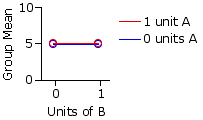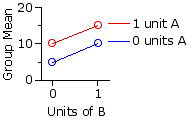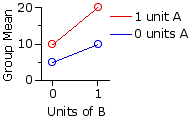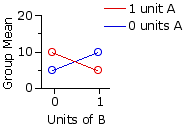Chapter 16.
Two-Way Analysis of Variance for Independent Samples
Part 1

As indicated in Chapter 13, the two-way ANOVA is a procedure that examines the effects of two independent variables concurrently. This not only provides a kind of economy in allowing you to look at two things for the price of one. It also, and often much more importantly, allows you to determine whether the two independent variables interact with respect to their effect on the dependent variable.

I will introduce this critically important concept with the familiar example of drug interactions. Suppose we were interested in the effects of two drugs, A and B, on the blood level of a certain hormone. To this end, we randomly and independently sort the members of our subject pool (healthy adult human males between the ages of 30 and 40) into four equal-sized groups. As shown in the following table, group r1c1 ("r"=row, "c"=column) serves as a kind of control group, receiving only an inert placebo containing zero units of A and zero units of B. Group r1c2 receives zero units of A and 1 unit of B; r2c1 receives 1 unit of A and zero units of B; and r2c2 receives 1 unit each of A and B.
 Note that the data structure in the two-variable case takes the form of a rows-by-columns matrix. For economy of expression, it is conventional to speak of the "row variable" and the "column variable" in accordance with how the two independent variables are arrayed in the matrix. In the present example, drug A is the row variable and drug B is the column variable.
 Drug B 0 units 1 unit DrugA 0units r1c10 units of A 0 units of B r1c20 units of A 1 unit of B 1unit r2c11 unit of A 0 units of B r2c21 unit of A 1 unit of B

The following tables and graphs show four possible scenarios. The numbers inside the blue cells in the table represent the respective mean blood levels of the hormone for each of the four groups following adminstration of the experimental treatment; the numbers to the right of each row and at the bottom of each column represent the mean for that particular row or column; and the number in the bottom right corner is the mean of the entire array. The adjacent graph in each scenario is a plot of the group mean values appearing in the blue cells.

Scenario 1|
 Drug B0 units 1 unit DrugA 0units 5 5 5 1unit 5 5 5 5 5 5

One need not know much about statistical inference to surmise that the two drugs in this scenario show no effect at all, either separately or in combination. This is what would be expected on the basis of the null hypothesis.

Scenario 2|
 Drug B0 units 1 unit DrugA 0units 5 10 7.5 1unit 10 15 12.5 7.5 12.5 10

In this scenario both drugs appear to increase the level of the hormone, but note that their combined effect is one of simple addition. With zero units of A and B, the mean blood level of the hormone is 5. Add 1 unit of B and it increases to 10. Start again from zero, add 1 unit of A, and it also increases from 5 to 10. When presented alone, 1 unit of either A or B increases the level of the hormone by 5. When presented together, they increase it by 5+5 to 15. The effect of the two drugs in combination is merely the sum of the effects they have separately.
 In considering the effects of the two independent variables separately, what the two-way ANOVA actually looks at are the differences among the means of the row variable and the differences among the means of the column variable. By convention, these two sets of differences are spoken of as the "main effects" of the analysis, as distinguished from the "interaction effect," which is something above and beyond the two main effects. Thus, for the present scenario, the main effect for the row variable, drug A, is the difference between Mrow1=7.5 and Mrow2=12.5. The main effect for the column variable, drug B, is the difference between Mcol1=7.5 and Mcol2=12.5. (In real life you will not normally get such a symmetrical assortment of row and column means.) In Scenario 1 there are no effects at all. In Scenario 2 there are main effects for A and B, but no interaction effect.

Scenario 3|
 Drug B0 units 1 unit DrugA 0units 5 10 7.5 1unit 10 20 15 7.5 15 11.25

Here the drugs when presented separately are having the same effects as in Scenario 2, but now they are also interacting. The effect of the two when presented in combination is greater than the sum of their separate effects.
 Note again that the main effects would consist of the difference between Mrow1=7.5 and Mrow2=15, and of the difference between Mcol1=7.5 and Mcol2=15. In this scenario there are main effects for rows and columns as well as an interaction effect.

Scenario 4|
 Drug B0 units 1 unit DrugA 0units 5 10 7.5 1unit 10 5 7.5 7.5 7.5 7.5

Here, too, is an interaction effect, but in the opposite direction. In Scenario 3 combining the drugs enhances their separate effects. Now the combined effect is one of mutual cancellation.
 But note that in this scenario there would be no main effects for either rows or columns (Mrow1=Mrow2=7.5 and Mcol1=Mcol2=7.5), notwithstanding that drugs A and B are obviously having an effect when presented separately. The moral of this story is that the interpretation of the presence or absence of main effects in a two-way ANOVA is not always simple and straightforward. More of this later.

These are by no means the only sorts of interaction effects that can be found, though I think they will give you at least a preliminary idea of what the concept is pointing to. Whenever two variables in combination produce effects that are different from the simple sum of their separate effects, you have the makings of an interaction. When represented graphically, the absence of an interaction will appear as lines that are approximately parallel, as in Scenario 2, while the presence of an interaction will appear as lines that diverge or converge or cross over each other, as in Scenarios 3 and 4.

¶Procedure

Up to a point, the two-way ANOVA for independent samples proceeds exactly like the corresponding one-way ANOVA. Here as well, SSbg is the measure of the aggregate differences among the several groups, and SSwg is the measure of random variability inside the groups. The latter, SSwg, is treated the same way as in the one-way analysis: it ends up as the MSerror that appears in the denominator of the F-ratio. But now, with two independent variables and the data arrayed in the form of a rows-by-columns matrix, SSbg can be further divided, like Caesar's Gaul and Freud's psychical topography, into three parts. As illustrated in the following diagram, one of these parts measures the differences among the means of the two or more rows, another measures the mean differences among the two or more columns, and the third is a measure of the degree to which the two independent variables interact. Each of these three components is then converted into a corresponding value of MS, with the result that there are now three separate F-ratios to calculate and three separate tests of significance to perform: one for the row variable, one for the column variable, and one for the interaction between the two variables.The two-way ANOVA is sufficiently important to warrant illustration with several examples. The first two are variations on the simple drug A versus drug B scenarios we have just examined. The third will be a bit more complex.

End of Chapter 16, Part 1.